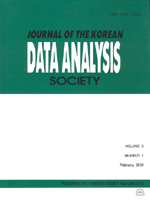상세검색
최근 검색어 전체 삭제
다국어입력
즐겨찾기0KCI등재 학술저널

# Modified Moriguti-Bulmer’s Method on Confidence Intervals of Variance in One-Factor Components-of-Variance Model

• 등재여부 : KCI등재
• 2009.12
• 2943 - 2954 (12 pages)

The confidence intervals for σ²A are given in various forms by many authors in one-factor components-of-variance model yij=μ+Ai+e ij. In this model, Moriguti(1954)-Bulmer’s(1957) method confidence intervals for σ²A are given by an asymptotic confidence intervals. By the simulation results of this confidence coefficients are not fit exactly for the given confidence coefficients and J is too large to use in the simulation. Thus, we would like to give one of the modified Moriguti(1954)-Bulmer’s(1957) method confidence intervals for σ²A in one-factor components-of-variance model. The simulation results, this modified confidence coefficients are better than those obtained by using Moriguti(1954)-Bulmer’s (1957) method confidence coefficients, and we can simulate when J is small.

1. Introduction

2. One-Factor Components-of-Variance Model

3. Modified Confidence Intervals

4. Simulation Results

References